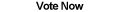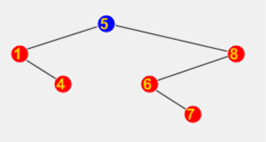Home Articles Components Code Snippets Links# C# Binary Search Tree## Binary Search Tree

A Binary Search Tree in C# is a data structure designed for fast searching through ordered values. A binary tree in computer science is very powerful and is the basis for more advanced data structures.

A Binary Search Tree consists of single nodes linked together. Each node is linked to at most two other "child" nodes. What makes a binary search tree unique is that for any given node, all nodes in the left subtree have values that are smaller (or equal) to the given node. Consequently values on the right subtree are bigger.

Thus searching through a Binary Tree is fast because only one of two paths down the tree can be taken when searching for a value.

## Inserting Values

Inserting a value into a Binary Search Tree is very simple. Start at the root of the tree (the top-most node). If the value to insert is smaller, go to the left child node. If the value is bigger then go to the right child. (If the value is the same, it's up to you to choose which side to store equal values).

Once it's decided to go to the left or right subtree, check if there's a node or if that subtree is "empty" (parent did not have a child there). If there was no child there, that is where the value is inserted. If there is an existing child there, repeat the same steps above.

Notice that a value inserted will always be a leaf in the tree. A leaf is a node that has no children nodes.

## Searching a Binary Tree

Searching through a Binary Tree is exactly like inserting a value. However instead of looking for an "empty" spot to place the value, you want to match the value of a node with the parameter value.

If after making the proper left-right decisions, it reaches an empty node, it can be safely assumed that the value does not exist within the Binary Search Tree.

## Removing Nodes

Removing a value from a Binary Tree consists of 3 cases.

The first case is that the node of the value is a leaf. This is the easiest case because the node only needs to be removed from the parent.

The second case is that the node has one child. To remove the node, replace the node with its only child node. (That is, make the child node's new parent be the original node's parent. Consequently update the child reference of the parent to the new child).

The third case is that the node has two children. There are several ways to handle the removal, here is one way. Find the rightmost node in the left subtree of the original node (the inorder predecessor). Replace the value of the original node with the value of the inorder predecessor. Then delete the inorder predecessor with one of the two cases above. (Because it is the rightmost node, it can only be a leaf or have one child in the left subtree).

Something to remember when removing nodes is to update all the necessary references. Which includes updating which node is the root of the tree.

## Binary Tree Traversal

A tree traversal means iterating through every value in the Binary Tree. Since a tree consists of three components: the parent, the left child, and the right child, there are three ways to traverse through a Binary Tree.

The first way is an inorder traversal. An inorder traversal visits the left child first (recursively in case it has children nodes of its own), then visists the parent node, and visits the right child last.

The second way is a preorder traversal. A preorder traversal visits the parent node first, then left child, and then the right child.

The third way is a postorder traversal. A postorder traversal visits the left child first, then the right child, and then the parent node.

All three types of traversals are useful for different cases. For example, an inorder traversal will give you all the values in the Binary Search Tree in increasing order. While a postorder traversal is best when the goal is to remove all the nodes of the tree. Since there is no way to tell which traversal will be needed, the C# Binary Search Tree at the bottom of the page has a property to set which traversal mode to use.

## Notes on Binary Search Tree

A Binary Search Tree has equal running times for searching and inserting, in theory. In pratice a Binary Search Tree can become unbalanced. An unbalanced Binary Tree is one that does not have an even distribution of children. For example, try inserting the values 0-10 in order into a Binary Search Tree. Since the values are inserting in increasing order, they are always inserted on the right subtree. At the end you end up with what looks like a LinkedList.

More complex data structures have been devised to create self-balancing Binary Trees in .NET, for example the AVL Tree and Red-Black Tree..

Back to C# Article List

Other C# Articles